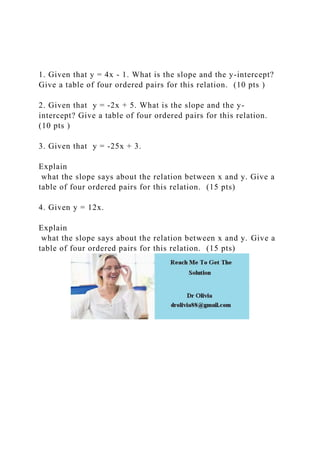Seu SlideShare está sendo baixado. ×

# 1. Given that y = 4x - 1. What is the slope and the y-intercept.docx

Anúncio
Anúncio
Anúncio
Anúncio
Anúncio
Anúncio
Anúncio
Anúncio
Anúncio
Anúncio
AnúncioCarregando em…3
×

1 de 1 Anúncio

# 1. Given that y = 4x - 1. What is the slope and the y-intercept.docx

1. Given that y = 4x - 1. What is the slope and the y-intercept? Give a table of four ordered pairs for this relation.  (10 pts )

2. Given that  y = -2x + 5. What is the slope and the y-intercept? Give a table of four ordered pairs for this relation.  (10 pts )

3. Given that  y = -25x + 3.

Explain
what the slope says about the relation between x and y. Give a table of four ordered pairs for this relation.  (15 pts)

4. Given y = 12x.

Explain
what the slope says about the relation between x and y. Give a table of four ordered pairs for this relation.  (15 pts)
.

1. Given that y = 4x - 1. What is the slope and the y-intercept? Give a table of four ordered pairs for this relation.  (10 pts )

2. Given that  y = -2x + 5. What is the slope and the y-intercept? Give a table of four ordered pairs for this relation.  (10 pts )

3. Given that  y = -25x + 3.

Explain
what the slope says about the relation between x and y. Give a table of four ordered pairs for this relation.  (15 pts)

4. Given y = 12x.

Explain
what the slope says about the relation between x and y. Give a table of four ordered pairs for this relation.  (15 pts)
.

Anúncio
Anúncio

### 1. Given that y = 4x - 1. What is the slope and the y-intercept.docx

1. 1. 1. Given that y = 4x - 1. What is the slope and the y-intercept? Give a table of four ordered pairs for this relation. (10 pts ) 2. Given that y = -2x + 5. What is the slope and the y- intercept? Give a table of four ordered pairs for this relation. (10 pts ) 3. Given that y = -25x + 3. Explain what the slope says about the relation between x and y. Give a table of four ordered pairs for this relation. (15 pts) 4. Given y = 12x. Explain what the slope says about the relation between x and y. Give a table of four ordered pairs for this relation. (15 pts)